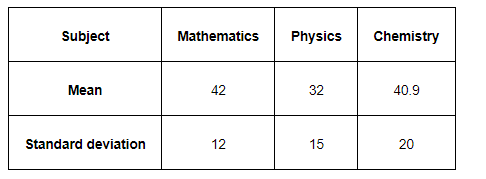# The mean and standard deviation of marks obtained by 50 students of a class in three subjects,

Question:

The mean and standard deviation of marks obtained by 50 students of a class in three subjects, Mathematics, Physics and Chemistry are given below:Which of the three subjects shows the highest variability in marks and which shows the lowest?

Solution:

Standard deviation of Mathematics = 12

Standard deviation of Physics = 15

Standard deviation of Chemistry = 20

The coefficient of variation (C.V.) is given by $\frac{\text { Standard deviation }}{\text { Mean }} \times 100$.

C.V. (in Mathematics) $=\frac{12}{42} \times 100=28.57$

C.V. $($ in Physics $)=\frac{15}{32} \times 100=46.87$

C.V.(in Chemistry) $=\frac{20}{40.9} \times 100=48.89$

The subject with greater C.V. is more variable than others.

Therefore, the highest variability in marks is in Chemistry and the lowest variability in marks is in Mathematics.